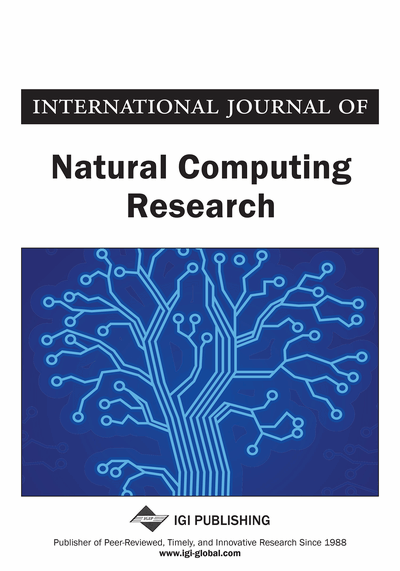# Multi-Objective Evolutionary Algorithm NSGA-II for Variables Selection in Multivariate Calibration Problems

Daniel Vitor de Lucena (Informatics Institute, Universidade Federal de Goiás (UFG), Goiânia, Brazil), Telma Woerle de Lima (Informatics Institute, Universidade Federal de Goiás (UFG), Goiânia, Brazil), Anderson da Silva Soares (Informatics Institute, Universidade Federal de Goiás (UFG), Goiânia, Brazil) and Clarimar José Coelho (Departament of Computation, Pontifícia Universidade Católica de Goiás, Goiânia, Brazil)
DOI: 10.4018/jncr.2012100103

## Abstract

This paper proposes a multiobjective formulation for variable selection in multivariate calibration problems in order to improve the generalization ability of the calibration model. The authors applied this proposed formulation in the multiobjective genetic algorithm NSGA-II. The formulation consists in two conflicting objectives: minimize the prediction error and minimize the number of selected variables for multiple linear regression. These objectives are conflicting because, when the number of variables is reduced the prediction error increases. As study of case is used the wheat data set obtained by NIR spectrometry with the objective for determining a variable subgroup with information about protein concentration. The results of traditional techniques of multivariate calibration as the partial least square and successive projection algorithm for multiple linear regression are presented for comparisons. The obtained results showed that the proposed approach obtained better results when compared with a mono-objective evolutionary algorithm and with traditional techniques of multivariate calibration.
Article Preview
Top

## 1. Introduction

The chemmometrics is a branch of analytical chemistry that uses knowledge mathematical, statistical, and logic to develop methods to chemical data analysis (Brown, Blank, Sum, & Weyer, 1994; Yusoff, Venkat, Yusof, & Abdullah, 2012). The main goal this area is the concentration determination of analyte collected using instrumental methods (Beebe, Pell & Seasholtz, 1998). The concentration value is obtained indirectly from direct measurements (absortion, light emission) made by the instrument using a calibration model that relates the physical measurements with the concentration of interest analyte (Skoog, 2008).

Prediction in chemmometrics is a procedure that use a multivariate model to predict the properties of a given sample. The absorbance at a wavelength can be related to the concentration of an analyte (Martens, 1989). The multivariate calibration is related to the construction of a mathematic model to calculate a predict valuebased on measured values of a set of explanatory variablesThere are popular calibration models to building multivariate regression model as Multiple Linear Regression (MLR) (Martens, 1989), Principal Component Regression (PCR) (Jolliffe, 1982) and Partial Least Square Regression (PLSR) (Beebe, et al., 1998; Martens & Naes, 1989).

Sometimes, it isn’t necessary the use of all collected data of a sample during the calibration process to analyze just some features of the sample. The selection of variables with information related to these features of interest allows creating more parsimonious and simple models, which are also easy of interpretation (Gaspar-Cunha, Mendes, Duarte, Vieira, Ribeiro, Ribeiro, & Neves, 2010). Others problems also found on calibration are the collinearity and sensitivity. The collinearity happens when two or more variables have correlated information. The sensitivity to noise prejudice the calibration efficiency and prediction of the compounds of sample, in particular MLR models (Martens & Naes, 1989; Draper, Smith, & Pownell, 1966).

A solution to the collinear variables is to obliterate them through variable selection methods (Guyon, & Elisseeff, 2003). At this process, the use of evolutionary algorithms, in particular Genetic Algorithms (GAs) are promising methods. An optimization algorithm like an evolutionary algorithm can be used to choose a strong subset of variables with little redundancy and information related to the characteristics of interest (Holland, 1992).

At this work we propose the use of the multi-objective genetic algorithms NSGA-II to the variables selection process. This problem has two conflicting objectives: minimize the residual error between concentration predicted by the MLR model and the real protein concentration of the grain, and minimize the number of selected variables. When we reduce the number of selected variables we also reduce the computational cost and simplify the calibration model (Coello, Lamont, & Van Veldhuisen, 2007).

## Complete Article List

Search this Journal:
Reset
Open Access Articles: Forthcoming
Volume 9: 4 Issues (2020): 1 Released, 3 Forthcoming
Volume 8: 4 Issues (2019)
Volume 7: 4 Issues (2018)
Volume 6: 2 Issues (2017)
Volume 5: 4 Issues (2015)
Volume 4: 4 Issues (2014)
Volume 3: 4 Issues (2012)
Volume 2: 4 Issues (2011)
Volume 1: 4 Issues (2010)
View Complete Journal Contents Listing